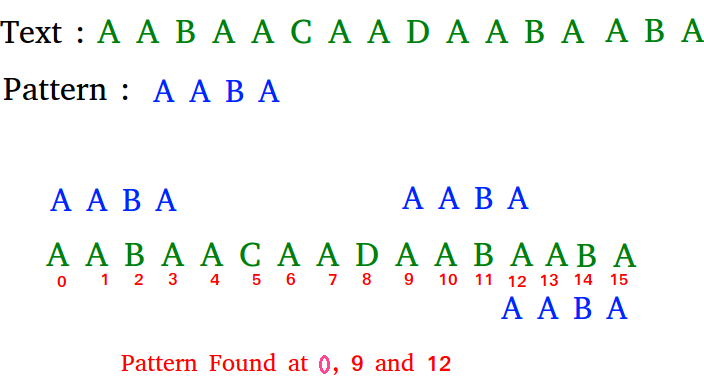# Python Program for KMP Algorithm for Pattern Searching

• Difficulty Level : Easy
• Last Updated : 08 Jun, 2022

Given a text txt[0..n-1] and a pattern pat[0..m-1], write a function search(char pat[], char txt[]) that prints all occurrences of pat[] in txt[]. You may assume that n > m
Examples:

```Input:  txt[] = "THIS IS A TEST TEXT"
pat[] = "TEST"
Output: Pattern found at index 10

Input:  txt[] =  "AABAACAADAABAABA"
pat[] =  "AABA"
Output: Pattern found at index 0
Pattern found at index 9
Pattern found at index 12```` `

Pattern searching is an important problem in computer science. When we do search for a string in notepad/word file or browser or database, pattern searching algorithms are used to show the search results.

## Python3

 `# Python program for KMP Algorithm``def` `KMPSearch(pat, txt):``    ``M ``=` `len``(pat)``    ``N ``=` `len``(txt)` `    ``# create lps[] that will hold the longest prefix suffix``    ``# values for pattern``    ``lps ``=` `[``0``]``*``M``    ``j ``=` `0` `# index for pat[]` `    ``# Preprocess the pattern (calculate lps[] array)``    ``computeLPSArray(pat, M, lps)` `    ``i ``=` `0` `# index for txt[]``    ``while` `i < N:``        ``if` `pat[j] ``=``=` `txt[i]:``            ``i ``+``=` `1``            ``j ``+``=` `1` `        ``if` `j ``=``=` `M:``            ``print` `(``"Found pattern at index"``, ``str``(i``-``j))``            ``j ``=` `lps[j``-``1``]` `        ``# mismatch after j matches``        ``elif` `i < N ``and` `pat[j] !``=` `txt[i]:``            ``# Do not match lps[0..lps[j-1]] characters,``            ``# they will match anyway``            ``if` `j !``=` `0``:``                ``j ``=` `lps[j``-``1``]``            ``else``:``                ``i ``+``=` `1` `def` `computeLPSArray(pat, M, lps):``    ``len` `=` `0` `# length of the previous longest prefix suffix` `    ``lps[``0``] ``# lps is always 0``    ``i ``=` `1` `    ``# the loop calculates lps[i] for i = 1 to M-1``    ``while` `i < M:``        ``if` `pat[i]``=``=` `pat[``len``]:``            ``len` `+``=` `1``            ``lps[i] ``=` `len``            ``i ``+``=` `1``        ``else``:``            ``# This is tricky. Consider the example.``            ``# AAACAAAA and i = 7. The idea is similar``            ``# to search step.``            ``if` `len` `!``=` `0``:``                ``len` `=` `lps[``len``-``1``]` `                ``# Also, note that we do not increment i here``            ``else``:``                ``lps[i] ``=` `0``                ``i ``+``=` `1` `txt ``=` `"ABABDABACDABABCABAB"``pat ``=` `"ABABCABAB"``KMPSearch(pat, txt)` `# This code is contributed by Bhavya Jain`

Output:

`Found pattern at index 10`

Time Complexity: O(m+n)

Space Complexity: O(m)

Please refer complete article on KMP Algorithm for Pattern Searching for more details!

My Personal Notes arrow_drop_up